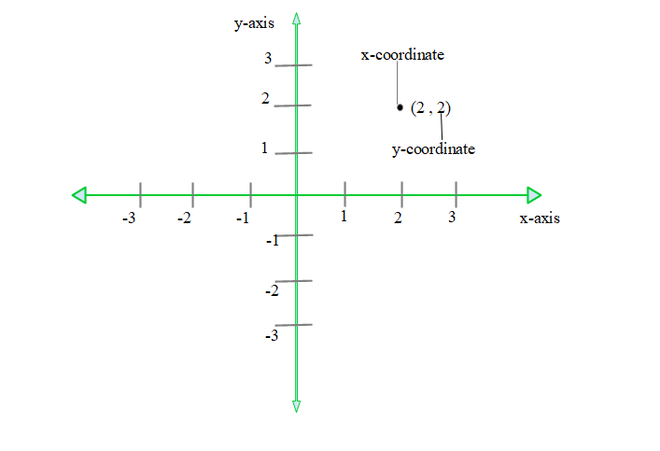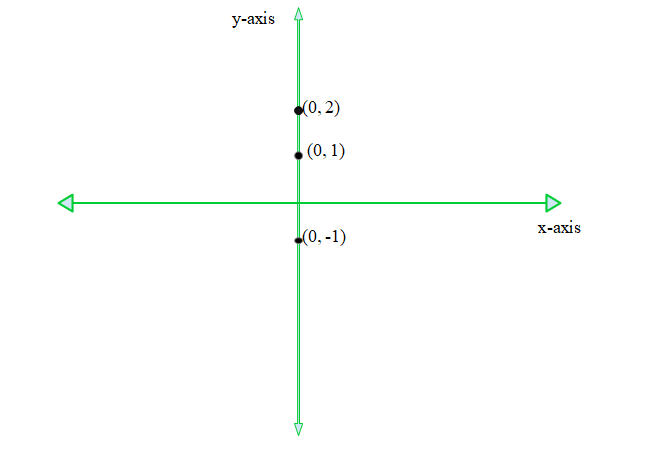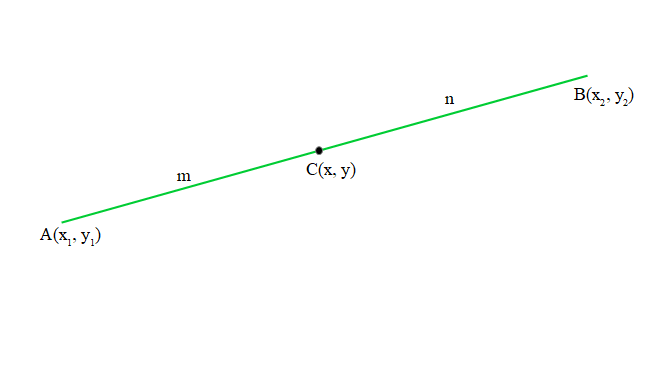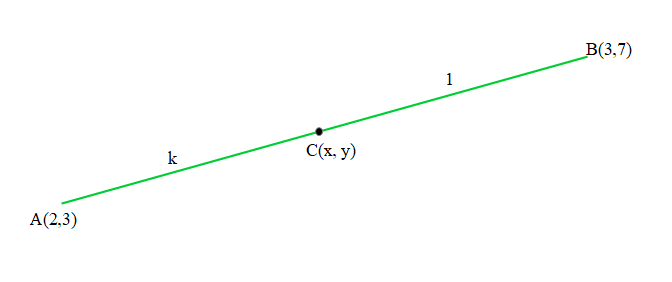# In what ratio is the line segment joining the points (2, 3) and (3, 7) divided by Y-axis?

• Last Updated : 02 Dec, 2021

Mathematics is a subject that is associated with numbers and calculations. And, according to the type of calculation mathematics is divided into different branches like algebra, geometry, arithmetic, etc. Geometry is the branch of mathematics that deals with shape and their properties. Geometry that deals with a point, lines, and planes in which coordinates are involved is called coordinate geometry.

### Coordinates

The location of any point on a plane can be expressed as (x, y) and these pairs are known as the coordinates, x is the horizontal value of a point on the plane. This value can also be called the x-coordinate or Abscissa, y is the vertical value of a point on the plane. This value can be called the y-coordinate or ordinate. In coordinate geometry, the point is represented on the cartesian plane.Cartesian plane

Divided by y-axis means the point which lies on the y-axis.The point which lies on the y-axis will always have its x-coordinate to be zero.

### Section formula

If a line AB has coordinates (x1, y1) and (x2, y2) of point A and B respectively and there is a point C on line AB which divides the line in ratio m:n, then the coordinate of C can be obtained from below formula, which is known as section formula:1. x = (mx2 + nx1) /( m + n)
2. y = (my2 + ny1) / (m + n)

### In what ratio is the line segment joining the points (2, 3) and (3, 7) divided by Y-axis?

Solution:

In the above problem statement, there is one line segment say AB having coordinates (2,3) and (3,7) of A and B respectively. Let’s consider a point C having coordinate (x, y) which lies on the y-axis, then the x coordinate of point C is 0, the coordinates of C can be written as (0, y).

Find the ratio in which this C point will divide the line segment AB. To find the ratio, use the section formula of coordinate geometry. Let’s assume that point C divides AB into a k:1 ratio.As known, the x-coordinate of point C, we can use it to obtain the value of k and get the ratio. Here,

m = k, n =1

x1 = 2, y1 = 3

x2 = 3, y2 = 7

x- coordinate using section formula:

x = (mx2 + nx1) / (m + n)

After substituting the values,

x = ((k)(3) + (1)(2))/ (k +1)

0 = (3k + 2)/ (k + 1)

0 = 3k + 2

– 2 = 3k

-2/3 = k

C divides the point in ratio k:1 = -2/3:1, therefore ratio is 2:3

A negative sign represents that point C divides the line segment externally in ratio 2:3.

### Sample Problems

Question 1: In what ratio is the line segment joining the points A and B have coordinates (0, -2) and (3, 1) respectively divided by X-axis?

Solution:

Let C be the point on X-axis that divides AB in ratio k: 1. As C lies on the x-axis, the y-coordinate of the C should be 0. Therefore, the coordinate of C : (x, 0). As known as the y-coordinate of point C, we can use it to obtain the value of k and get the ratio. Here,

m = k, n = 1

x1 = 0, y1 = -2

x2 = 3, y2 = 1

y- coordinate using section formula:

y = (my2 + ny1) / (m + n)

After substituting the values,

y = ((k)(1) + (1)(-2))/ (k +1)

0 = ( k – 2)/ (k + 1)

0 = k – 2

2 = k

2 = k

C divides the point in ratio k:1 = 2:1, therefore ratio is 2:1.

Question 2: In what ratio does a point (2,2) divides the line segment AB of which (0, 0) and (5, 5) are the coordinates of A and B respectively.

Solution:

Let (2, 2) divide AB in ratio k: 1. As known the a and y-coordinate of point, use it to obtain the value of k and get the ratio. Here,

m = k, n = 1

x1 = 0, y1 = 0

x2 = 5, y2 = 5

y- coordinate using section formula:

y = (my2 + ny1) / (m + n)

After substituting the values,

2 = ((k)(5) + (1)(0)) / (k + 1)

2 = ( 5k ) / (k+1)

2(k + 1) = 5k

2k + 2 = 5k

2 = 3k

2/3 = k

Hence, (0, 0 ) divides the point in ratio k:1 = 2/3:1, therefore ratio is 2:3.

My Personal Notes arrow_drop_up
Recommended Articles
Page :